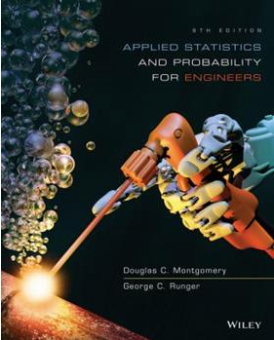LearnChemE#### Applied Statistics and Probability for Engineers (6th Ed.)

##### D.C. Montgomery and G.C. Runger
###### 3 Discrete Random Variables and Probability Distributions
3.6 Binomial Distribution
3.8 Hypergeometric Distribution
###### 5 Joint Probability Distributions
5.1 Two or More Random Variables
###### 7 Point Estimation of Parameters and Sampling Distributions
7.2 Sampling Distributions and the Central Limit Theorem
###### 8 Statistical Intervals for a Single Sample
8.1 Confidence Interval on the Mean of a Normal Distribution, Variance Known
8.2 Confidence Interval on the Mean of a Normal Distribution, Variance Unknown
8.5 Guidelines for Constructing Confidence Intervals
###### 9 Tests of Hypotheses for a Single Sample
9.1 Hypothesis Testing
9.2 Tests on the Mean of a Normal Distribution, Variance Known
###### 10 Statistical Inference for Two Samples
10.2 Inference on the Difference in Means of two Normal Distributions, Variances Unknown
10.6 Inference on Two Population Proportions
###### 12 Multiple Linear Regression
12.1 Multiple Linear Regression Model
12.2 Hypothesis Tests in Multiple Linear Regression
###### 13 Design and Analysis of Single-Factor Experiments: The Analysis of Variance
13.2 Completely Randomized Single-Factor Experiment
###### 14 Design of Experiments with Several Factors
14.3 Two-Factor Factorial Experiments
14.6 Blocking and Confounding in the 2^k Design
14.7 Fractional Replication of the 2k Design